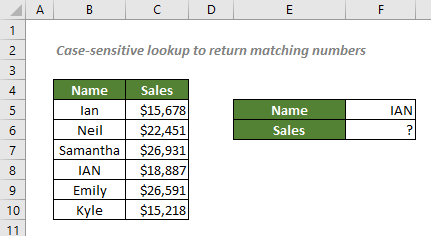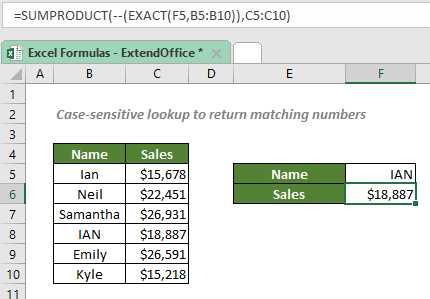## Case-sensitive lookup to return matching numbers

From our previous tutorial Case-Sensitive Lookup, you may know that with the help of EXACT function, you can combine the INDEX and MATCH functions, or use the VLOOKUP function to perform a case-sensitive lookup. However, if you need to lookup numeric values only, you can just use the combination of the SUMPRODUCT and EXACT functions to easily get the result you want.#### How to perform a case-sensitive lookup to return matching numbers?

To find out the sales made by IAN as shown in the screenshot above, you can use the EXACT function to compare the text strings in the name range to the specific name IAN, the value in the cell F5, including the case of each character. The EXACT will generate an array, and which will be fed to the SUMPRODUCT function to sum the elements of the array to get IAN’s sales.

#### Generic syntax

=SUMPRODUCT(--(EXACT("lookup_value",lookup_range)),return_range)

√ Note: The values in the return_range should be numeric values.

• lookup_value: The value EXACT used to perform a case-sensitive comparison with the text strings in the lookup_range. Here refers to the given name, IAN.
• lookup_range: The range of cells to compare with the lookup_value. Here refers to the name range.
• return_range: The range where you want the combination formula to return the numeric value from. Here refers to the sales range.

To find out the sales made by IAN, please copy or enter the formula below in the cell F6, and press Enter to get the result:

=SUMPRODUCT(--(EXACT("IAN",B5:B10)),C5:C10)

Or, use a cell reference to make the formula dynamic:

=SUMPRODUCT(--(EXACT(F5,B5:B10)),C5:C10)#### Explanation of the formula

=SUMPRODUCT(--(EXACT(F5,B5:B10)),C5:C10)

• --(EXACT(F5,B5:B10)): The EXACT function compares the text strings in the name range B5:B10 against "IAN", the value in the cell F5, and returns a TRUE if a value in the cells through B5 to B10 is exactly the same as IAN, a FALSE otherwise. So, we will get an array of TRUEs and FALSEs like this:
{FALSE;FALSE;FALSE;TRUE;FALSE;FALSE}
The double negative, i.e. --, converts the TRUEs and FALSEs to 1s and 0s like this:
{0;0;0;1;0;0}.
• SUMPRODUCT(--(EXACT(F5,B5:B10)),C5:C10) = SUMPRODUCT({0;0;0;1;0;0},{15678;22451;26931;18887;26591;15218}): The SUMPRODUCT function multiplies the items of these two arrays in the same positions, and produce an array like this: {0;0;0;18887;0;0}. Then the function sums all the numeric values and returns 18887.

#### Related functions

Excel SUMPRODUCT function

In Excel, the SUMPRODUCT function can be used to multiply two or more columns or arrays together, and then get the sum of products. In fact, the SUMPRODUCT is a useful function which can help to count or sum cell values with multiple criteria like the COUNTIFS or SUMIFS function. This article will introduce the function syntax and some examples for this SUMPRODUCT function.

Excel EXACT function

The EXACT function compares two strings and returns TRUE if they are exactly same (taking into account case sensitivity), or returns FALSE.

#### Related Formulas

Case-Sensitive Lookup

You may know that you can combine the INDEX and MATCH functions, or use the VLOOKUP function to lookup values in Excel. However, the lookups aren’t case-sensitive. So, in order to perform a case-sensitive match, you should take advantage of the EXACT and CHOOSE functions.

Approximate match with INDEX and MATCH

There are times when we need to find approximate matches in Excel to evaluate employees' performance, grade students’ scores, calculate postage based on weight, etc. In this tutorial, we will talk about how to use the INDEX and MATCH functions to retrieve the results we need.

Lookup closest match value with multiple criteria

In some cases, you may need to lookup the closest or approximate match value based on more than one criteria. With the combination of INDEX, MATCH and IF functions, you can quickly get it done in Excel.

### The Best Office Productivity Tools

#### Kutools for Excel - Helps You To Stand Out From Crowd

 Popular Features: Find, Highlight or Identify Duplicates  |  Delete Blank Rows  |  Combine Columns or Cells without Losing Data  |  Round without Formula ... Super VLookup: Multiple Criteria  |  Multiple Value  |  Across Multi-Sheets  |  Fuzzy Lookup... Adv. Drop-down List: Easy Drop Down List  |  Dependent Drop Down List  |  Multi-select Drop Down List... Column Manager: Add a Specific Number of Columns  |  Move Columns  |  Toggle Visibility Status of Hidden Columns  |  Compare Columns to Select Same & Different Cells ... Featured Features: Grid Focus  |  Design View  |  Big Formula Bar  |  Workbook & Sheet Manager | Resource Library (Auto Text)  |  Date Picker  |  Combine Worksheets  |  Encrypt/Decrypt Cells  |  Send Emails by List  |  Super Filter  |  Special Filter (filter bold/italic/strikethrough...) ... Top 15 Toolsets:  12 Text Tools (Add Text, Remove Characters ...)  |  50+ Chart Types (Gantt Chart ...)  |  40+ Practical Formulas (Calculate age based on birthday ...)  |  19 Insertion Tools (Insert QR Code, Insert Picture from Path ...)  |  12 Conversion Tools (Numbers to Words, Currency Conversion ...)  |  7 Merge & Split Tools (Advanced Combine Rows, Split Excel Cells ...)  |  ... and more

Kutools for Excel Boasts Over 300 Features, Ensuring That What You Need is Just A Click Away...#### Office Tab - Enable Tabbed Reading and Editing in Microsoft Office (include Excel)

• One second to switch between dozens of open documents!
• Reduce hundreds of mouse clicks for you every day, say goodbye to mouse hand.
• Increases your productivity by 50% when viewing and editing multiple documents.
• Brings Efficient Tabs to Office (include Excel), Just Like Chrome, Edge and Firefox.